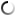# Friday, August 12, 2011

Square square1, square2, square3, square4;

int maxAttempts;

int attempts;

boolean done;

void setup()
{
size(400, 400);

square1 = new Square();

square1.x = 10;
square1.y = 10;

square1.r = 255;
square1.g = 0;
square1.b = 0;

square2 = new Square();
square2.r = 0;
square2.g = 255;
square2.b = 0;

square2.x = 100;
square2.y = 10;

square3 = new Square();
square3.r = 0;
square3.g = 0;
square3.b = 255;

square3.x = 100;
square3.y = 100;

square4 = new Square();

square4.x = 80;
square4.y = 0;

square4.r = 255;
square4.g = 255;
square4.b = 0;

// not used now
maxAttempts = 5;
attempts = 0;
done = false;
}

void draw()
{
background(100);

if (frameCount > 90)
{
square1.x = 900; // off the screen
}

square1.draw();
square2.draw();
square3.draw();
square4.draw();
}

void mousePressed()
{
if ( square1.isOver() )
{
println("Square 1 pressed");
}

if ( square2.isOver() )
{
println("Square 2 pressed");
}

if ( square3.isOver() )
{
println("Square 3 pressed");
}

if ( square4.isOver() )
{
println("Square 4 pressed");
}

}

// this is our square class
class Square
{
// width in pixels
int w;

int x;
int y;

// colors
int r;
int g;
int b;

boolean over;

// this is the "constructor" for a new Square
Square()
{
x = 0;
y = 0;
w = 50;
r = 0;
g = 0;
b = 0;
over = false;
}

void draw()
{

// set the fill color for the 1st square
if (over)
{
fill(255);
}
else
{
fill( r, g, b );
}

// draw the rectangle
rect(x, y, w, w);
}

boolean isOver()
{
if ( (mouseX > x) && (mouseX < x+w) &&
(mouseY > y) && (mouseY < y+w))
{
over = true;
}
else
{
over = false;
}

return over;
}

}

## commentsloading...